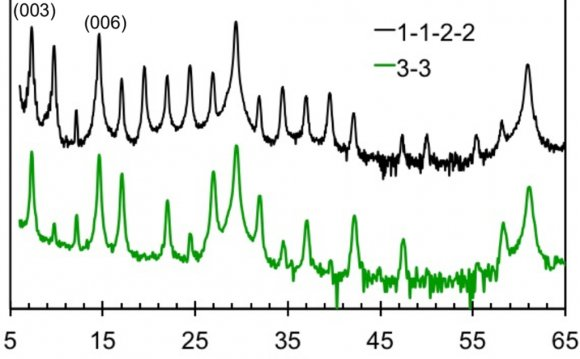# Diffraction Maxima

January 30, 2023### Diffraction and interference

• Outcome of diffraction is not simple. Diffraction leads to intersecting rays of light, which are coherent and interfere.
• Interference pattern formed by the diffracted light is called, for short diffraction pattern
• Sometimes it is emphasized that we speak about diffraction picture when we consider interference from many sources - as for example continous distribution of sources between the barriers in the Figure above.
• Note: Diffraction and interference are different phenomena (despite what textbook says :) )

### Single slit diffraction

• One of the most important examples of diffraction effects is the passage of light through a hole of finite size.
• If light passes through narrow, point like hole, almost exact spherical wave forms. However if the hole has a finite size, an interference pattern forms behind the hole
• We shall consider narrow (but finite width) slits, rather than round holes. The wavefronts that are formed by the slit are cyllindical rather than spherical
Single Slit Diffraction Applet
• Note that the interference patern of diffracted light from a wide slit although has geometrical similarity to two narrow slits, is guite different from as far as intensity distribution is concerned (see for example much brighter central fringe)
• We shall compute the distribution of the intensity at the screen beyond the slit.
• The pattern near the slit, where exact wavefront profiles are important are called near-field, or Fresnel, diffraction . The pattern at the screen far away, where we can use geometrical optics rays and approximations we have done in intereference studies is called far-field, or Fraunhofer diffraction
• We shall consider exclusively the far-field, Fraunhofer, diffraction pattern.

### Minima of Intensity in Fraunhofer diffraction pattern from a single slit

• This is simple, minima is achived at observation angles θ where sin(π(a/λ) sinθ) vanishes, i.e sin(θmin) = ±m λ/a
• but not for m = 0 , only for m = 1, 2 ...
• The separation between minima widens when the wavelengths increases or the slit width decreases
• This is why when slit is very narrow a ≈ 0 , the first minima is far away and one has continous distribution of light.
• sinθ varies from -1 to with zero achieved straight ahead of the slit. Thus, the number of minima observed is nmin = 2 int(a/λ)
• If slit is narrower than the wavelength, a < λ, no minima are observed.

### Maxima of Intensity in Fraunhofer diffraction pattern from a single slit

• The main maxima of intensity is at θ = 0 (yes, zero divide by zero gives one here ! )
• Because main maximum is where minimum is expected to be for m=0, it is twice wider than other maxima, occuping space between minima at ± λ/a . It's width in terms of sinθ is 2 λ/a
• Positions of other maxima are only approximately halfway between the minima sin(θmax) ≈ ± (m + ½) λ/a
• Depending on we speak about first order (m=±1), second order (m=±2) etc. maxima
• The approximate value of peak intensity at the maximum m > 0 is then Im ≈ I0 / ( π2 (m + ½)2)
Source: www.ualberta.ca
##### RELATED VIDEOdiffraction_intro.mp47. Physics | Diffraction of Light | Diffraction Maxima due ...